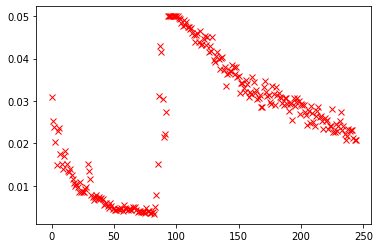# Awkward behavior of loss

I have written my own implementation of GRU. Which is

``````class new_meaning(NN.Module):
def __init__(self,input_size, hidden_size, output_size):
super(new_meaning,self).__init__()
self.hn = NN.Linear(hidden_size, hidden_size)
self.inp = NN.Linear(input_size, input_size)
self.reset_gate = NN.Linear(input_size + hidden_size, hidden_size )
self.update_gate = NN.Linear(input_size + hidden_size, hidden_size )
self.tan_h = NN.Linear(input_size + hidden_size, hidden_size )
self.linear = NN.Linear(hidden_size, hidden_size)
self.conclude = NN.Linear(hidden_size, vector_size)

def forward(self,inputs, first_hid, lengths = -1):
this_hid = first_hid
gru_out = []
for i in range(21):
this_hid = self.gru_forward(inputs[:,i,:] ,this_hid)
gru_out.append(this_hid)
gru_out = torch.stack(gru_out, dim = 1)
last_ones = torch.stack([gru_out[i,j,:] for (i,j) in lengths])
final_out = self.linear(last_ones)
conclusion = self.conclude(final_out)
return conclusion

def gru_forward(self, inputs, hidden):
concat = torch.cat((inputs,hidden), dim = 1)
reset = torch.sigmoid(self.reset_gate(concat))
update = torch.sigmoid(self.update_gate(concat))
temp_hid = reset*self.hn(hidden)
new_hid = torch.cat((temp_hid, self.inp(inputs)), dim = 1)
h_tilda = torch.tanh(self.tan_h(new_hid))
next_hid_1 = (1-update)*hidden
next_hid_2 = update*h_tilda
return next_hid_1 + next_hid_2

def init_hidden(self, batch_size = 1):
The problem I am facing is that it is not converging while using default GRU of Pytorch is converging. Also the losses initially seem to converge and then start behaving erratically.